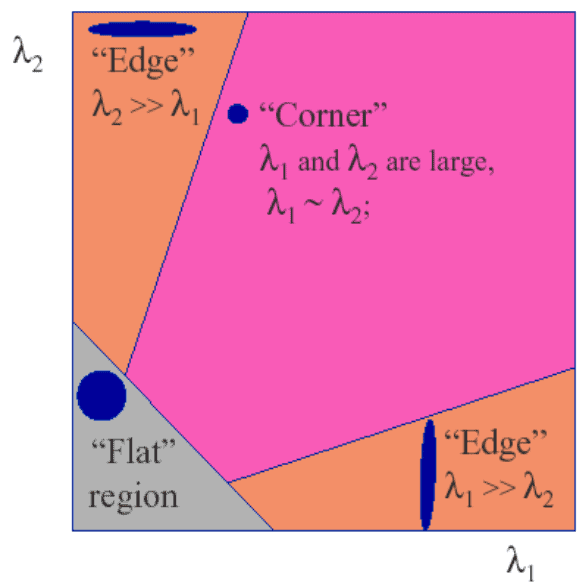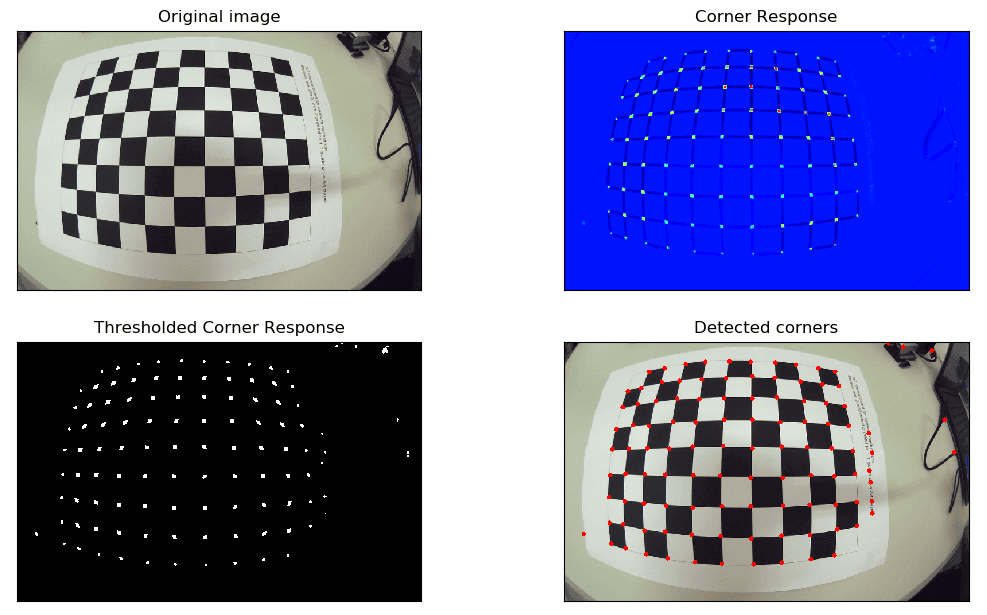## 1. Overview

In this tutorial, we’ll talk about Harris Corner Detection, a mathematical approach to detecting corners in an image. First, we’ll illustrate the mathematical formulation. Then, we’ll show the step-by-step procedure to perform the Harris Corner Detector.

## 2. Mathematical Formulation

A corner is a point whose local neighborhood is characterized by large intensity variation in all directions. Corners are important features in computer vision because they are points stable over changes of viewpoint and illumination. The so-called Harris Corner Detector was introduced by Chris Harris and Mike Stephens in 1988 in the paper “A Combined Corner and Edge Detector”.

Let’s consider a two-dimensional imageand a patchof sizecentered in. We want to evaluate the intensity variation occurred if the windowis shifted by a small amount. Such variation can be estimated by computing the Sum of Squared Differences (SSD):

(1)whereis a window function that can be a rectangular or a Gaussian function. We need to maximize the functionfor corner detection. Sinceandare small, the shifted intensitycan be approximated by the following first-order Taylor expansion:

(2)whereandare partial derivatives ofinanddirection, respectively. By substituting (2) in (1) we obtain:

(3)The equation (3) can be expressed in the following matrix form:

(4)whereis amatrix computed from image derivatives:

(5)The matrixis called structure tensor.

The Harris detector uses the following response function that scores the presence of a corner within the patch:

(6)is a constant to chose in the range. Sinceis a symmetric matrix,andwhereandare the eigenvalues of. Hence, we can express the corner response as a function of the eigenvalues of the structure tensor:

(7)So the eigenvalues determine whether a region is an edge, a corner or flat:

• ifandare small, thenis small and the region is flat;
• ifor viceversa, thenand the region is an edge;
• ifand both eigenvalues are large, then R is large and the region is a corner.

The classification of the points using the eigenvalues of the structure tensor is represented in the following figure:## 3. Algorithm

Harris corner detector consists of the following steps.

1. Convert the original image into a grayscale image. The pixel values ofare computed as a weighted sum of the corresponding,,values:

(8)2. Compute the derivativesandby convolving the imagewith the Sobel operator:

(9)3. Compute the products of the derivatives,,.
4. Convolve the images,,with a Gaussian filter or a mean filter. Define the structure tensor for each pixel as expressed in eq. (5).
5. Compute the response function for each pixel:

(10)6. Set a thresholdon the value ofand find pixels with responses above this threshold. Finally, compute the non-max suppression in order to pick up the optimal corners.

## 4. Example

In the following figure we show a real application of the Harris Corner Detector. The corner response map (top-right image) was computed using Eq. 10 with k=0.04. The bottom-left image represents the thresholded corner response obtained with. The detected corners obtained after the application of the non-max suppression are shown in the bottom-right image.## 5. Conclusion

In this article, we reviewed the Harris Corner Detector, a fundamental technique in computer vision. We explained the mathematical formulation, and finally, we explained how to implement it.